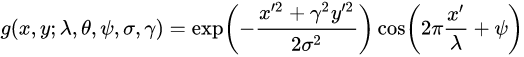# #StackBounty: #neural-networks #optimization #gradient-descent #convolution #filter What is wrong with my approach on a custom way of c…

### Bounty: 100

Disclosure: I am not a prominent mathematician (current bachelor student) like others on this website and my approach has been mostly pragmatic. Please do tell me if I can improve the formulation of my question and/or should provide more background information.

For the past few weeks I have been investigating in what I feel like is a smart way of improving on the way the first convolution layer of a neural network is created.
In the ‘vanilla’ way, the convolution kernels are simply initialized randomly and the kernel ‘weights’ are being tweaked respectively through back-propagation.

In a fairly recent blog post by Distill Pub, in which they investigate the shape and behaviour of early convolution kernels of various popular neural networks, it is found that rougly 44% of the initial kernels are very similar to Gabor filters.
My idea is to constrain the ‘hypothesis space’ of the first convolution layer in such a network to just be Gabor filters where the neural network may tweak the parameters of the Gabor filter rather than the raw kernel ‘weights’.

The formula I use for (real valued) Gabor filters is as follows:where $$x’ = xcos(theta) + ysin(theta)$$ and $$y’ = -xsin(theta) + ycos(theta)$$

I have spent quite a lot of time on implementing this idea, however I cannot get the gradient descent to work properly. I suspect there is something fundamentally wrong with my approach, which is why I am turning to the experts.

My current experiment setup Currently, I have an input image that I convolve with a predefined (hardcoded) kernel, with theta set to $$pi/4$$ (the other variables may be considered constant) to produce an output $$x$$. This makes it so that I can see what kernel would yield output $$x$$.
Next, I initialize the Gabor filter to be updated with a theta of $$- pi / 4$$, which I expect to be corrected to the actual value of $$pi/4$$. At the start of the training loop, when convolved with the image the convolution does not yield input $$x$$.
Through back propagation the $$theta$$ parameter should now be tweaked in order to obtain output $$x$$. I am currently using a flavor of RMSE, but this should not be important.

Results
In actuality, this is not what happens at all. The $$theta$$ parameter is being updated, albeit in a sensible direction (as measured by the loss value), at some point is jumps back to having a
terrible loss value and this cycle seems to continue indefinitely. I have tried implementing Momentum without any better results.

My main suspicion are the cycling components of the partial derivative of the Gabor function, which I have let Wolfram Alpha compute for me here. Furthermore, could it be that the Gabor function does not have a nice reachable minimum with respect to $$theta$$.

Now to my overarching question. Where does this break down? Does the partial derivative just not posses the right properties for it to find a proper global minimum? Did I simply make a mistake in the code, since this should theoretically be possible? How do I proceed to debug my approach?

Note: I have omitted any code here, as I am more interested in the theoretical feasibility of my approach rather than an implementation.

Get this bounty!!!

This site uses Akismet to reduce spam. Learn how your comment data is processed.